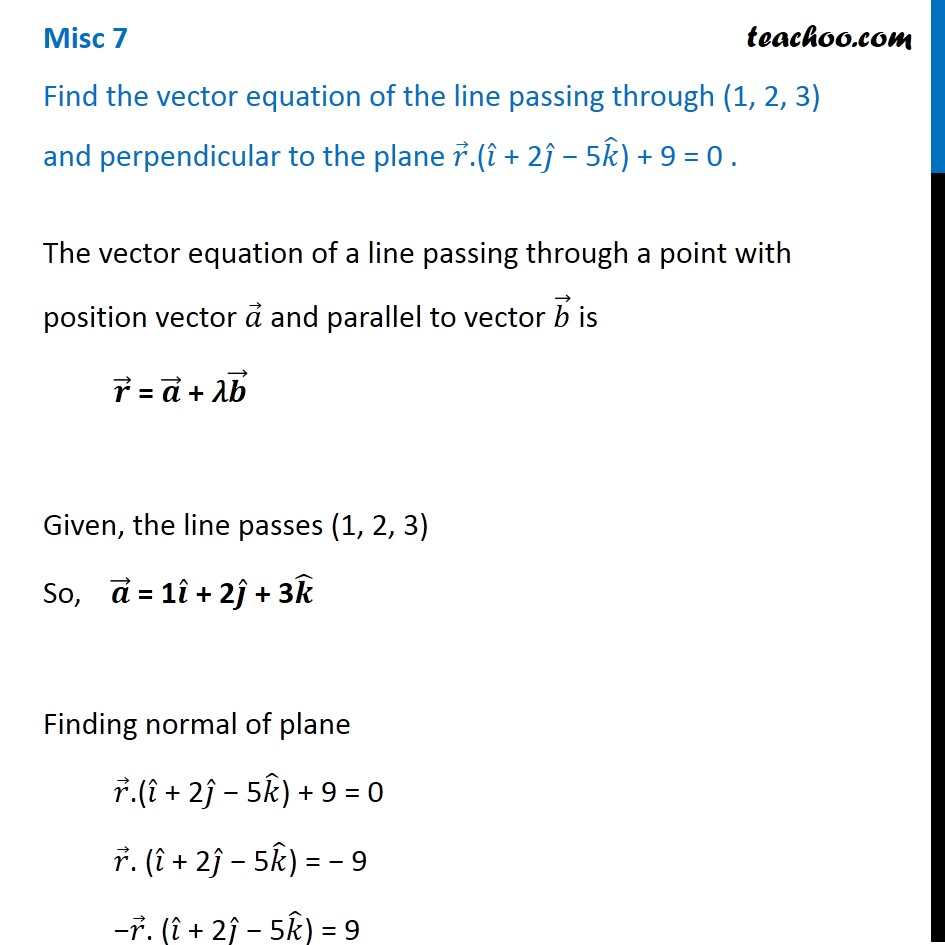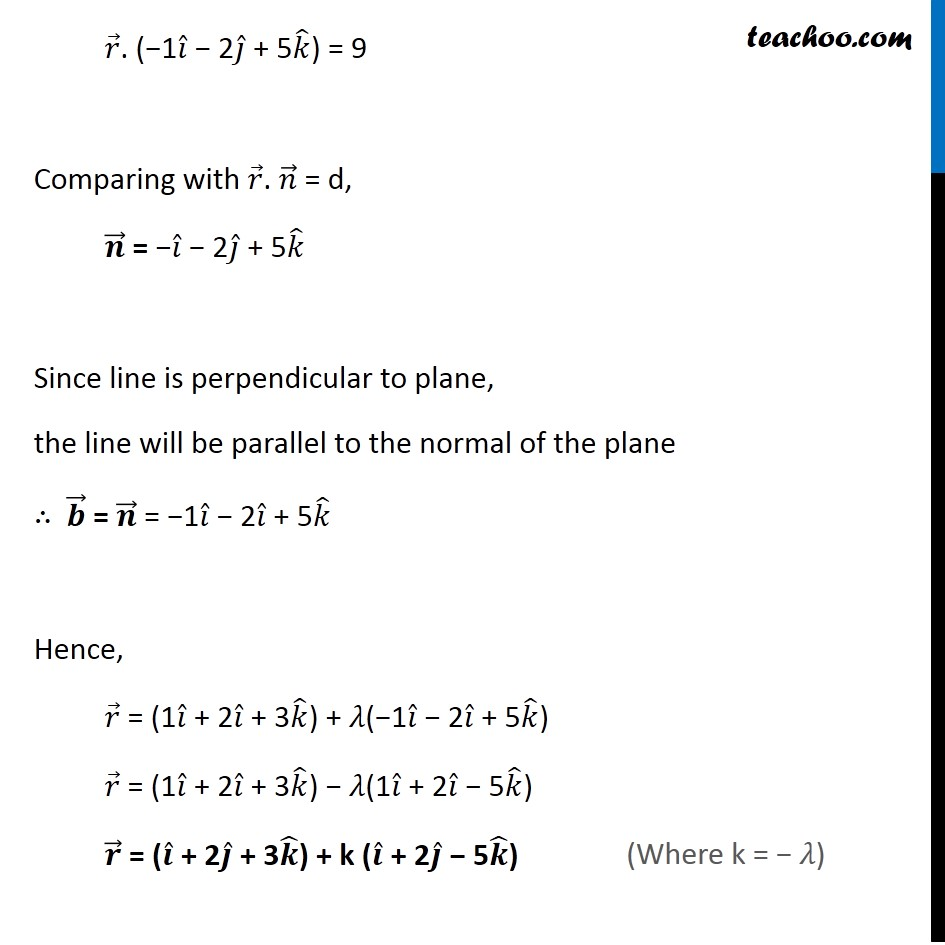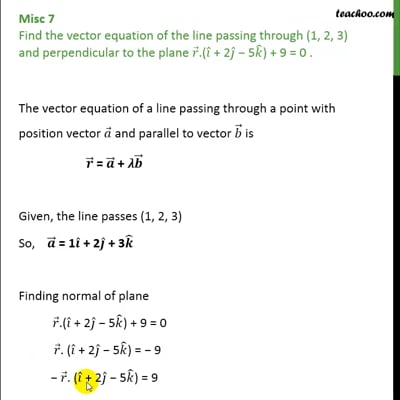Miscellaneous

Chapter 11 Class 12 Three Dimensional Geometry
Serial order wiseThis video is only available for Teachoo black users

Introducing your new favourite teacher - Teachoo Black, at only ₹83 per month

### Transcript

Misc 7 Find the vector equation of the line passing through (1, 2, 3) and perpendicular to the plane 𝑟 ⃗.(𝑖 ̂ + 2𝑗 ̂ − 5𝑘 ̂) + 9 = 0 . The vector equation of a line passing through a point with position vector 𝑎 ⃗ and parallel to vector 𝑏 ⃗ is 𝒓 ⃗ = 𝒂 ⃗ + 𝜆𝒃 ⃗ Given, the line passes (1, 2, 3) So, 𝒂 ⃗ = 1𝒊 ̂ + 2𝒋 ̂ + 3𝒌 ̂ Finding normal of plane 𝑟 ⃗.(𝑖 ̂ + 2𝑗 ̂ − 5𝑘 ̂) + 9 = 0 𝑟 ⃗. (𝑖 ̂ + 2𝑗 ̂ − 5𝑘 ̂) = − 9 −𝑟 ⃗. (𝑖 ̂ + 2𝑗 ̂ − 5𝑘 ̂) = 9 𝑟 ⃗. (−1𝑖 ̂ − 2𝑗 ̂ + 5𝑘 ̂) = 9 Comparing with 𝑟 ⃗. 𝑛 ⃗ = d, 𝒏 ⃗ = −𝑖 ̂ − 2𝑗 ̂ + 5𝑘 ̂ Since line is perpendicular to plane, the line will be parallel to the normal of the plane ∴ 𝒃 ⃗ = 𝒏 ⃗ = −1𝑖 ̂ − 2𝑖 ̂ + 5𝑘 ̂ Hence, 𝑟 ⃗ = (1𝑖 ̂ + 2𝑖 ̂ + 3𝑘 ̂) + 𝜆(−1𝑖 ̂ − 2𝑖 ̂ + 5𝑘 ̂) 𝑟 ⃗ = (1𝑖 ̂ + 2𝑖 ̂ + 3𝑘 ̂) − 𝜆(1𝑖 ̂ + 2𝑖 ̂ − 5𝑘 ̂) 𝒓 ⃗ = (𝒊 ̂ + 2𝒋 ̂ + 3𝒌 ̂) + k (𝒊 ̂ + 2𝒋 ̂ − 5𝒌 ̂)